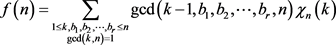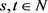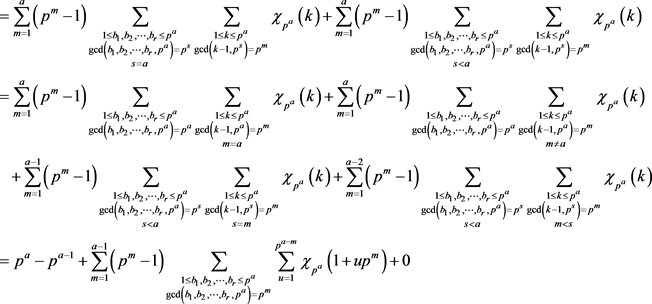﻿ 带有一个狄利克雷特征的Menon-Sury恒等式

# 带有一个狄利克雷特征的Menon-Sury恒等式Menon-Sury’s Identity with a Dirichlet Character

Li, Hu和Kim运用整数剩余类环及其单位群的滤链证明了Menon-Sury恒等式的如下推广：,

Abstract:
Li, Hu and Kim  proved the following generalization of the Menon-Sury identity by using the filtrations of the ring   Znand its unit group Z*n:,
where φ is Euler’s Totient function,, and χ is a Dirichlet character mod n with conductor d. In this paper, we re-prove the above identity based the orthogonality of Dirichlet characters and elementary calculations .

Abstract:

1. 引言

1965年，P.K. Menon  发现了下面这个漂亮的恒等式

$\underset{\begin{array}{c}1\le k\le n\\ \mathrm{gcd}\left(k,n\right)=1\end{array}}{\sum }\mathrm{gcd}\left(k-1,n\right)=\phi \left(n\right)\tau \left(n\right)$ (1)

$\underset{\begin{array}{c}1\le k,{b}_{1},{b}_{2},\cdots ,{b}_{r}\le n\\ \mathrm{gcd}\left(k,n\right)=1\end{array}}{\sum }\mathrm{gcd}\left(k-1,{b}_{1},{b}_{2},\cdots ,{b}_{r},n\right)=\phi \left(n\right){\sigma }_{r}\left(n\right)$ (2)

$\underset{\begin{array}{c}1\le k\le n\\ \mathrm{gcd}\left(k,n\right)=1\end{array}}{\sum }\mathrm{gcd}\left(k-1,n\right)\chi \left(k\right)=\phi \left(n\right)\tau \left(\frac{n}{d}\right)$ (3)

$\underset{\begin{array}{c}1\le k,{b}_{1},{b}_{2},\cdots ,{b}_{r}\le n\\ \mathrm{gcd}\left(k,n\right)=1\end{array}}{\sum }\mathrm{gcd}\left(k-1,{b}_{1},{b}_{2},\cdots ,{b}_{r},n\right)\chi \left(k\right)=\phi \left(n\right)$

$\underset{\begin{array}{c}1\le k,{b}_{1},{b}_{2},\cdots ,{b}_{r}\le n\\ \mathrm{gcd}\left(k,n\right)=1\end{array}}{\sum }\mathrm{gcd}\left(k-1,{b}_{1},{b}_{2},\cdots ,{b}_{r},n\right)\chi \left(k\right)=\phi \left(n\right){\sigma }_{r}\left(\frac{n}{d}\right)$

2. 定理1.1的证明

$\underset{u=1}{\overset{{p}^{a-m}}{\sum }}\chi \left(1+u{p}^{m}\right)=0$

$\begin{array}{l}f:{\left(Z/{p}^{a}Z\right)}^{*}\to {\left(Z/{p}^{m}Z\right)}^{*}\\ \text{ }\text{ }\text{ }\text{ }\text{\hspace{0.17em}}\text{\hspace{0.17em}}\text{\hspace{0.17em}}\stackrel{¯}{a}↦\stackrel{¯}{a}\end{array}$

$\text{#}\mathrm{ker}f=\frac{\phi \left({p}^{a}\right)}{\phi \left({p}^{m}\right)}=\frac{{p}^{a-1}\left(p-1\right)}{{p}^{m-1}\left(p-1\right)}={p}^{a-m}=#U$

${\left(Z/{p}^{a}Z\right)}^{*}/\mathrm{ker}f\cong {\left(Z/{p}^{m}Z\right)}^{*}$

$\underset{s\in U}{\sum }\chi \left(s\right)=0=\underset{u=1}{\overset{{p}^{a-m}}{\sum }}\chi \left(1+u{p}^{m}\right)$

$\underset{\begin{array}{c}1\le k,{b}_{1},{b}_{2},\cdots ,{b}_{r}\le {p}^{a}\\ \mathrm{gcd}\left({b}_{1},{b}_{2},\cdots ,{b}_{r},{p}^{a}\right)={p}^{m}\end{array}}{\sum }1={\left({p}^{a-m}\right)}^{r}-{\left({p}^{a-m-1}\right)}^{r}$

$\underset{\begin{array}{c}1\le k\le {p}^{n-m}\\ \mathrm{gcd}\left(k,p\right)=1\end{array}}{\sum }\chi \left(k{p}^{m}+1\right)=\left\{\begin{array}{l}0,\text{ }\text{ }1\le m

$\underset{\begin{array}{c}1\le k\le {p}^{n-m}\\ \mathrm{gcd}\left(k,p\right)=1\end{array}}{\sum }\chi \left(k{p}^{m}+1\right)=\left\{\begin{array}{l}\phi \left({p}^{n-m}\right),\text{ }\text{ }l\le m。其中 ${\chi }_{n}$ 为一个模n的狄利克雷特征，存在使得 $\mathrm{gcd}\left(s,t\right)=1$ ，且成立下面等式：

$\begin{array}{l}f\left(st\right)=\underset{\begin{array}{c}1\le k,{b}_{1},{b}_{2},\cdots ,{b}_{r}\le st\\ \mathrm{gcd}\left(k,st\right)=1\end{array}}{\sum }\mathrm{gcd}\left(k-1,{b}_{1},{b}_{2},\cdots ,{b}_{r},st\right){\chi }_{st}\left(k\right)\\ =\underset{\begin{array}{c}1\le {k}_{1},{b}_{1},{b}_{2},\cdots ,{b}_{r}\le s\\ \mathrm{gcd}\left({k}_{1},s\right)=1\end{array}}{\sum }\underset{\begin{array}{c}1\le {k}_{2},{b}_{1},{b}_{2},\cdots ,{b}_{r}\le t\\ \mathrm{gcd}\left({k}_{2},t\right)=1\end{array}}{\sum }\mathrm{gcd}\left({k}_{1}t+{k}_{2}s-1,{b}_{1},{b}_{2},\cdots ,{b}_{r},st\right){\chi }_{s}\left({k}_{1}t+{k}_{2}s\right){\chi }_{t}\left({k}_{1}t+{k}_{2}s\right)\\ =\underset{\begin{array}{c}1\le {k}_{1},{b}_{1},{b}_{2},\cdots ,{b}_{r}\le s\\ \mathrm{gcd}\left({k}_{1},s\right)=1\end{array}}{\sum }\underset{\begin{array}{c}1\le {k}_{2},{b}_{1},{b}_{2},\cdots ,{b}_{r}\le t\\ \mathrm{gcd}\left({k}_{2},t\right)=1\end{array}}{\sum }\mathrm{gcd}\left({k}_{1}t-1,{b}_{1},{b}_{2},\cdots ,{b}_{r},s\right)\mathrm{gcd}\left({k}_{2}s-1,{b}_{1},{b}_{2},\cdots ,{b}_{r},t\right){\chi }_{s}\left({k}_{1}t\right){\chi }_{t}\left({k}_{2}s\right)\\ =f\left(s\right)f\left(t\right)\end{array}$ (4)

$\begin{array}{l}f\left({p}^{a}\right)=\underset{\begin{array}{c}1\le k,{b}_{1},{b}_{2},\cdots ,{b}_{r}\le {p}^{a}\\ \mathrm{gcd}\left(k,{p}^{a}\right)=1\end{array}}{\sum }\mathrm{gcd}\left(k-1,{b}_{1},{b}_{2},\cdots ,{b}_{r},{p}^{a}\right){\chi }_{{p}^{a}}\left(k\right)\\ =\underset{1\le k,{b}_{1},{b}_{2},\cdots ,{b}_{r}\le {p}^{a}}{\sum }\mathrm{gcd}\left(k-1,{b}_{1},{b}_{2},\cdots ,{b}_{r},{p}^{a}\right){\chi }_{{p}^{a}}\left(k\right)\\ =\underset{\begin{array}{c}1\le k,{b}_{1},{b}_{2},\cdots ,{b}_{r}\le {p}^{a}\\ \mathrm{gcd}\left(k-1,{b}_{1},{b}_{2},\cdots ,{b}_{r},{p}^{a}\right)\ne 1\end{array}}{\sum }\mathrm{gcd}\left(k-1,{b}_{1},{b}_{2},\cdots ,{b}_{r},{p}^{a}\right){\chi }_{{p}^{a}}\left(k\right)+\underset{\begin{array}{c}1\le k,{b}_{1},{b}_{2},\cdots ,{b}_{r}\le {p}^{a}\\ \mathrm{gcd}\left(k-1,{b}_{1},{b}_{2},\cdots ,{b}_{r},{p}^{a}\right)=1\end{array}}{\sum }{\chi }_{{p}^{a}}\left(k\right)\\ =\underset{m=1}{\overset{a}{\sum }}\underset{\begin{array}{c}1\le k,{b}_{1},{b}_{2},\cdots ,{b}_{r}\le {p}^{a}\\ \mathrm{gcd}\left(k-1,{b}_{1},{b}_{2},\cdots ,{b}_{r},{p}^{a}\right)={p}^{m}\end{array}}{\sum }{p}^{m}{\chi }_{{p}^{a}}\left(k\right)-\underset{m=1}{\overset{a}{\sum }}\underset{\begin{array}{c}1\le k,{b}_{1},{b}_{2},\cdots ,{b}_{r}\le {p}^{a}\\ \mathrm{gcd}\left(k-1,{b}_{1},{b}_{2},\cdots ,{b}_{r},{p}^{a}\right)={p}^{m}\end{array}}{\sum }{\chi }_{{p}^{a}}\left(k\right)+\underset{1\le {b}_{1},{b}_{2},\cdots ,{b}_{r}\le {p}^{a}}{\sum }\underset{1\le k\le {p}^{a}}{\sum }{\chi }_{{p}^{a}}\left(k\right)\\ =\underset{m=1}{\overset{a}{\sum }}\underset{\begin{array}{c}1\le k,{b}_{1},{b}_{2},\cdots ,{b}_{r}\le {p}^{a}\\ \mathrm{gcd}\left(k-1,{b}_{1},{b}_{2},\cdots ,{b}_{r},{p}^{a}\right)={p}^{m}\end{array}}{\sum }\left({p}^{m}-1\right){\chi }_{{p}^{a}}\left(k\right)+\underset{1\le {b}_{1},{b}_{2},\cdots ,{b}_{r}\le {p}^{a}}{\sum }0\end{array}$$f\left({p}^{a}\right)=\phi \left({p}^{a}\right)+\underset{m=1}{\overset{a-1}{\sum }}\left({p}^{m}-1\right)\underset{\begin{array}{c}1\le {b}_{1},{b}_{2},\cdots ,{b}_{r}\le {p}^{a}\\ \mathrm{gcd}\left({b}_{1},{b}_{2},\cdots ,{b}_{r},{p}^{a}\right)={p}^{m}\end{array}}{\sum }0=\phi \left({p}^{a}\right)$

3. 定理1.2的证明

$\underset{\begin{array}{c}1\le k,{b}_{1},{b}_{2},\cdots ,{b}_{r}\le {p}^{a}\\ \mathrm{gcd}\left(k,{p}^{a}\right)=1\end{array}}{\sum }\mathrm{gcd}\left(k-1,{b}_{1},{b}_{2},\cdots ,{b}_{r},{p}^{a}\right)\chi \left(k\right)=\phi \left({p}^{a}\right){\sigma }_{r}\left(\frac{{p}^{a}}{{p}^{l}}\right)$

$\begin{array}{l}\underset{\begin{array}{c}1\le k,{b}_{1},{b}_{2},\cdots ,{b}_{r}\le {p}^{a}\\ \mathrm{gcd}\left(k,{p}^{a}\right)=1\end{array}}{\sum }\mathrm{gcd}\left(k-1,{b}_{1},{b}_{2},\cdots ,{b}_{r},{p}^{a}\right){\chi }_{{p}^{a}}\left(k\right)\\ ={p}^{a}-1+\underset{m=1}{\overset{a-1}{\sum }}\left({p}^{m}-1\right)\underset{\begin{array}{c}1\le k\le {p}^{a}\\ \mathrm{gcd}\left(k-1,{p}^{a}\right)={p}^{m}\\ m\ne a\end{array}}{\sum }{\chi }_{{p}^{a}}\left(k\right)+\underset{m=1}{\overset{a-1}{\sum }}\left({p}^{m}-1\right)\underset{\begin{array}{c}1\le {b}_{1},{b}_{2},\cdots ,{b}_{r}\le {p}^{a}\\ \mathrm{gcd}\left({b}_{1},{b}_{2},\cdots ,{b}_{r},{p}^{a}\right)={p}^{m}\\ m

$\begin{array}{l}A={p}^{a}-1,\\ B=\underset{m=1}{\overset{a-1}{\sum }}\left({p}^{m}-1\right)\underset{\begin{array}{c}1\le k\le {p}^{a}\\ \mathrm{gcd}\left(k-1,{p}^{a}\right)={p}^{m}\\ m\ne a\end{array}}{\sum }{\chi }_{{p}^{a}}\left(k\right),\\ C=\underset{m=1}{\overset{a-1}{\sum }}\left({p}^{m}-1\right)\underset{\begin{array}{c}1\le {b}_{1},{b}_{2},\cdots ,{b}_{r}\le {p}^{a}\\ \mathrm{gcd}\left({b}_{1},{b}_{2},\cdots ,{b}_{r},{p}^{a}\right)={p}^{m}\\ m

$\begin{array}{c}B=\underset{m=1}{\overset{a-1}{\sum }}\left({p}^{m}-1\right)\underset{\begin{array}{c}1\le j\le {p}^{a-m}\\ \mathrm{gcd}\left(j,p\right)=1\end{array}}{\sum }{\chi }_{{p}^{a}}\left(1+j{p}^{m}\right)\\ =\underset{m=1}{\overset{l-1}{\sum }}\left({p}^{m}-1\right)\underset{\begin{array}{c}1\le j\le {p}^{a-m}\\ \mathrm{gcd}\left(j,p\right)=1\end{array}}{\sum }{\chi }_{{p}^{a}}\left(1+j{p}^{m}\right)+\underset{m=l}{\overset{a-1}{\sum }}\left({p}^{m}-1\right)\underset{\begin{array}{c}1\le j\le {p}^{a-m}\\ \mathrm{gcd}\left(j,p\right)=1\end{array}}{\sum }{\chi }_{{p}^{a}}\left(1+j{p}^{m}\right)\end{array}$

$=1-{p}^{a-1}+\left(a-l\right)\phi \left(pa\right)$

$\begin{array}{c}C=\underset{m=1}{\overset{a-1}{\sum }}\left({p}^{m}-1\right)\left({\left({p}^{a-m}\right)}^{r}-{\left({p}^{a-m-1}\right)}^{r}\right)\underset{1\le u\le {p}^{a-m}}{\sum }{\chi }_{{p}^{a}}\left(1+u{p}^{m}\right)\\ =\underset{m=1}{\overset{a-1}{\sum }}\left({p}^{m}-1\right)\left({\left({p}^{a-m}\right)}^{r}-{\left({p}^{a-m-1}\right)}^{r}\right)\underset{i=0}{\overset{a-m}{\sum }}\underset{\begin{array}{c}1\le v\le {p}^{a-m-i}\\ \text{gcd}\left(v,p\right)=1\end{array}}{\sum }{\chi }_{{p}^{a}}\left(1+v{p}^{m+i}\right)\\ =\underset{m=1}{\overset{a-1}{\sum }}\left({p}^{m}-1\right)\left({\left({p}^{a-m}\right)}^{r}-{\left({p}^{a-m-1}\right)}^{r}\right)\underset{i=0}{\overset{a-m}{\sum }}\underset{\begin{array}{c}1\le v\le {p}^{a-w}\\ \text{gcd}\left(v,p\right)=1\end{array}}{\sum }{\chi }_{{p}^{a}}\left(1+v{p}^{w}\right)\end{array}$

$\begin{array}{c}C=\underset{m=1}{\overset{l-2}{\sum }}\left({p}^{m}-1\right)\left({\left({p}^{a-m}\right)}^{r}-{\left({p}^{a-m-1}\right)}^{r}\right)\left(-{p}^{a-l}+\underset{i=l-m}{\overset{a-m-1}{\sum }}\phi \left({p}^{a-m-i}\right)+1\right)\\ \text{\hspace{0.17em}}\text{\hspace{0.17em}}+\left({p}^{l-1}-1\right)\left({\left({p}^{a-\left(l-1\right)}\right)}^{r}-{\left({p}^{a-\left(l-1\right)-1}\right)}^{r}\right)\left(-{p}^{a-l}+\underset{i=1}{\overset{a-l}{\sum }}\phi \left({p}^{a-\left(l-1\right)-i}\right)+1\right)\\ \text{\hspace{0.17em}}\text{\hspace{0.17em}}+\underset{m=l}{\overset{a-1}{\sum }}\left({p}^{m}-1\right)\left({\left({p}^{a-m}\right)}^{r}-{\left({p}^{a-m-1}\right)}^{r}\right)\left(\underset{i=0}{\overset{a-m-1}{\sum }}\phi \left({p}^{a-m-i}\right)+1\right)\\ =0+0+{p}^{a}\left({p}^{ar}-{p}^{ar-r}\right)\underset{m=l}{\overset{a-1}{\sum }}\left(1-{p}^{-m}\right){p}^{-mr}\\ ={p}^{ar+a-rl}-{p}^{a}-\frac{\left({p}^{1+r}-p\right)\left({p}^{ar+a-l-rl}-1\right)}{{p}^{1+r}-1}\end{array}$

$\begin{array}{c}D=\underset{m=1}{\overset{a-2}{\sum }}\left({p}^{m}-1\right)\underset{s=m+1}{\overset{a-1}{\sum }}\underset{\begin{array}{c}1\le {b}_{1},{b}_{2},\cdots ,{b}_{r}\le {p}^{a}\\ \mathrm{gcd}\left({b}_{1},{b}_{2},\cdots ,{b}_{r},{p}^{a}\right)={p}^{s}\end{array}}{\sum }\underset{\begin{array}{c}1\le j\le {p}^{a-m}\\ \mathrm{gcd}\left(j,p\right)=1\end{array}}{\sum }{\chi }_{{p}^{a}}\left(1+j{p}^{m}\right)\\ ={p}^{ar-r}\underset{m=1}{\overset{a-2}{\sum }}\left({p}^{m}-1\right){p}^{-mr}\left(1-{\left({p}^{-r}\right)}^{a-m-1}\right)\underset{\begin{array}{c}1\le j\le {p}^{a-m}\\ \mathrm{gcd}\left(j,p\right)=1\end{array}}{\sum }{\chi }_{{p}^{a}}\left(1+j{p}^{m}\right)\\ ={p}^{ar-r}\underset{m=1}{\overset{l-1}{\sum }}\left({p}^{m}-1\right){p}^{-mr}\left(1-{\left({p}^{-r}\right)}^{a-m-1}\right)\underset{\begin{array}{c}1\le j\le {p}^{a-m}\\ \mathrm{gcd}\left(j,p\right)=1\end{array}}{\sum }{\chi }_{{p}^{a}}\left(1+j{p}^{m}\right)\\ +{p}^{ar-r}\underset{m=l}{\overset{a-2}{\sum }}\left({p}^{m}-1\right){p}^{-mr}\left(1-{\left({p}^{-r}\right)}^{a-m-1}\right)\underset{\begin{array}{c}1\le j\le {p}^{a-m}\\ \mathrm{gcd}\left(j,p\right)=1\end{array}}{\sum }{\chi }_{{p}^{a}}\left(1+j{p}^{m}\right)\end{array}$

$\begin{array}{l}=\left({p}^{a-1}+{p}^{\left(r+1\right)\left(a-l\right)}-{p}^{ar+a-1-lr}-{p}^{a-l}\right)\\ \text{\hspace{0.17em}}\text{ }\text{ }\text{\hspace{0.17em}}+{p}^{ar-r}\phi \left({p}^{a}\right)\left(\underset{m=l}{\overset{a-2}{\sum }}{p}^{-mr}-\underset{m=l}{\overset{a-2}{\sum }}{p}^{r-ar}-\underset{m=l}{\overset{a-2}{\sum }}{p}^{\left(-r-1\right)m}+\underset{m=l}{\overset{a-2}{\sum }}{p}^{r-ar}{p}^{-m}\right)\\ ={p}^{a-1}-p+{p}^{ar+a-lr}\left({p}^{-l}-{p}^{-1}\right)-\left(a-l-1\right)\phi \left({p}^{a}\right)+\phi \left({p}^{a}\right)\frac{{p}^{ar-lr}-{p}^{r}}{{p}^{r}-1}\\ \text{\hspace{0.17em}}\text{\hspace{0.17em}}\text{ }\text{ }-\phi \left({p}^{a}\right)\frac{{p}^{ar-lr-l+1}-{p}^{r-a+2}}{{p}^{1+r}-1}\end{array}$

$A+B+C+D=\phi \left({P}^{a}\right){\sigma }_{r}\left(\frac{{p}^{a}}{{p}^{l}}\right)$ ,

$\begin{array}{l}\underset{\begin{array}{c}1\le k,{b}_{1},{b}_{2},\cdots ,{b}_{r}\le n\\ \mathrm{gcd}\left(k,n\right)=1\end{array}}{\sum }\mathrm{gcd}\left(k-1,{b}_{1},{b}_{2},\cdots ,{b}_{r},n\right)\chi \left(k\right)\\ =\underset{i=1}{\overset{r}{\prod }}\left(\underset{\begin{array}{c}1\le k,{b}_{1},{b}_{2},\cdots ,{b}_{r}\le n\\ \mathrm{gcd}\left(k,n\right)=1\end{array}}{\sum }\mathrm{gcd}\left(k-1,{b}_{1},{b}_{2},\cdots ,{b}_{r},n\right){\chi }_{{k}_{i}}\left(k\right)\right)\\ =\underset{i=1}{\overset{r}{\prod }}\left(\phi \left({p}_{i}^{a}\right){\sigma }_{r}\left(\frac{{p}_{i}^{a}}{{p}_{i}^{l}}\right)\right)\\ =\phi \left(n\right){\sigma }_{r}\left(nd\right)\end{array}$

 Li, Y., Hu, X. and Kim, D. (2018) A Generalization of Menon’s Identity with Dirichlet Characters. International Journal of Number Theory, 14, 2631-2639.
https://doi.org/10.1142/S1793042118501579

 Apostol, T.M. (1976) Introduction to Analytic Number Theory. Undergraduate Texts in Mathematics. Springer-Verlag, New York/Heidelberg

 Menon, P.K. (1965) On the Sum (a-1, n)[(a, n) = 1]. Journal of the Indian Mathematical Society, 29, 155-163.

 Sury, B. (2009) Some Number-Theoretic Identities from Group Actions. Rendiconti del Circolo Matematico di Palermo, 58, 99-108.
https://doi.org/10.1007/s12215-009-0010-6

 Miguel, C. (2014) Menon’s Identity in Residually Finite Dedekind Domains. Journal of Number Theory, 137, 179-185.
https://doi.org/10.1016/j.jnt.2013.11.003

 Miguel, C. (2016) A Menon-Type Identity in Residually Finite Dedekind Domains. Journal of Number Theory, 164, 43-51.
https://doi.org/10.1016/j.jnt.2015.12.018

 Zhao, X.-P. and Cao, Z.-F. (2017) Another Generalization of Menon’s Identity. International Journal of Number Theory, 13, 2373-2379.
https://doi.org/10.1142/S1793042117501299

Top